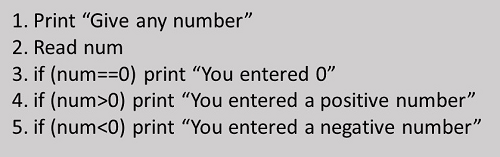# Writing the Algorithm

Advertisements

A finite set of steps that must be followed to solve any problem is called an algorithm. Algorithm is generally developed before the actual coding is done. It is written using English like language so that it is easily understandable even by non-programmers.

Sometimes algorithms are written using pseudocodes, i.e. a language similar to the programming language to be used. Writing algorithm for solving a problem offers these advantages −

• Promotes effective communication between team members

• Enables analysis of problem at hand

• Acts as blueprint for coding

• Assists in debugging

• Becomes part of software documentation for future reference during maintenance phase

These are the characteristics of a good and correct algorithm −

• Has a set of inputs

• Steps are uniquely defined

• Has finite number of steps

• Produces desired output

## Example Algorithms

Let us first take an example of a real-life situation for creating algorithm. Here is the algorithm for going to the market to purchase a pen.Step 4 in this algorithm is in itself a complete task and separate algorithm can be written for it. Let us now create an algorithm to check whether a number is positive or negative.Advertisements